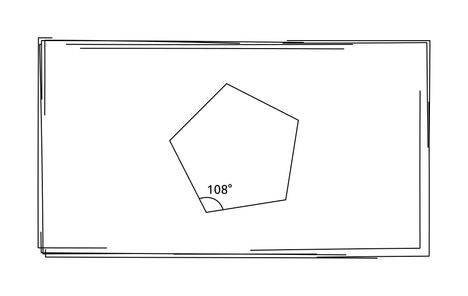# Practice on Toph

Participate in exhilarating programming contests, solve unique algorithm and data structure challenges and be a part of an awesome community.

# Angle of Polygon

Limits 1s, 512 MB

Nusrat is a brilliant student. She is good at all subjects but only get confused with geometry. Today her teacher gave some homework on geometry and the topic is regular polygon. Given the number of sides of a regular polygon, she needs to find out one of the interior angles of the polygon.

A regular polygon is a polygon that is equiangular (all angles are equal in measure) and equilateral (all sides have the same length). — Wikipedia

The following figure shows a regular polygon consisting of five sides in which one/each angle is 108°:As Nusrat is weak in geometry, she wants help from you.

## Input

The first line of the input will contain an integer T (0 < T ≤ 50), the number of test cases.

Each test case will contain a single integer N (3 ≤ N ≤ 100) in each line which denotes the number of sides of a regular polygon.

## Output

For each test case, print one interior angle of the corresponding polygon in a single line. The answer is considered correct if the absolute or relative error doesn’t exceed 10- 4.

## Sample

InputOutput
3
3
5
10
60.000000
108.000000
144.000000

•Geometry

### Statistics

94% Solution Ratio

SoudipEarliest, Sep '19

alif_biswasFastest, 0.0s

mahfuzAhmedLightest, 0 B

bokaifShortest, 48B

### Submit